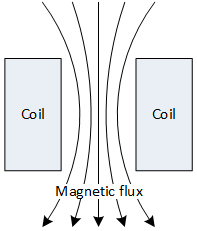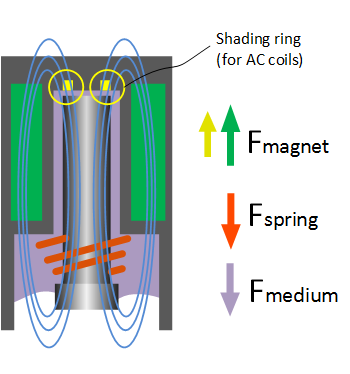# Understanding Solenoid Design & Function

## Electromagnetic controlSolenoid valves are actuated with the aid of a solenoid. This is basically an electric coil with a moveable core in the centre that is made from a ferromagnetic material. The core is often referred to as plunger. When current flows through the coil, a magnetic field is created around the coil. The strength of this field depends on the current, the number of windings and the material of the core. As a result of the magnetic field, the solenoid core is pulled towards the centre of the coil. As long as current is flowing, the core remains pulled towards the centre. As soon as the flow of current stops, the magnetic field disappears and the core (usually) is pushed down by a spring to its initial position.

The core, or plunger, should:

• have good magnetic properties
• have a low magnetic memory effect
• be wear resistant
• suitable to machine

## Force equilibrium of coil, spring and medium pressureThe basic principle of a solenoid valve is a force balance of the the magnetic force of the solenoid on one side, and the pressure of the medium and force of the spring on the other side. The required magnetic force for a direct operated solenoid valve can be calculated with the following formula:

Fs=p•A-Fspring

Where:

• Fs = solenoid force (N)
• p = pressure (Pa) (105 Pa = 1 bar)
• A = orifice (m2)
• Fspring = spring force (N)

### Example

A given solenoid provides a force of 15N. To use this solenoid to control a 10 bar pressure differential, the maximum orifice diameter can be calculated.

Fs=p•A → 15= 106•A
A = 1.5•10-5 m2
A = 1/4•π•d2 → d = 4.4 mm

Indirect operated solenoid valves have a smaller orifice and use the pressure of the medium to switch. This formula cannot be used for indirect operated valves.

## Solenoid valves for direct and alternating current

### Direct current

The power of the coil can be calculated with the following formula:

P= U2/R

where:

• P= electric power (W)
• U= voltage (V)
• R= elektrical resistance (I)

The properties of a coil for direct current are:

• No humming noise
• High solenoid force in lifted position
• Equal lifting force in initial and lifted position
• Energy consumption and magnetic force are dependent on temperature
• Less sensitive to dirt
• The coil has more windings than a coil for alternating current

### A myth

Often people think that the direction of the magnetic field in the coil determines the direct of force that acts on the core. This is not true. The core is ferromagnetic (and not a permanent magnet with defined poles), therefore the core will always be attracted to the centre of the magnetic field. Both coils for AC and DC pull the core towards the centre. It doesnt matter how the coil is connected to the power supply (the poles can be connected both ways).

### Alternating current

The electrical resistance of an AC coil is lower than the resistance of a DC coil at the same voltage. With AC coils, the current is not only determined by the resistance of the windings, but also by the inductive resistance (reactance). The inductive resistance is largely dependent by the position of the core. The further the core is removed from the coil, the lower the inductance resistance becomes. This means that the current through the coil increases. The current is lower when the core is in the lifted position. As a result, the coil should never be connected to the power supply when the core is removed. The coil can produce too much heat and burn in minutes.

The inductive reactance is not only determined by the position of the core, but also by the frequency of the current. The reactance is higher with 60Hz than with 50Hz. A 50Hz coil that is connected to a 60Hz supply, will have a lower magnetic force than specified. In the opposite case, when a 60Hz coil is connected to a 50Hz supply, the energy consumption will increase and the temperature of the coil will increase slightly.

Another important feature of AC coils is the use of a special conductive ring, called the shading ring, on the upper side of the coil. If it would not be used, the plunger would vibrate. Every time the voltage crosses the zero line, the magnetic force drops to zero. This happens twice per period. As a result, the plunger will move back and forth with a frequency of twice the mains frequency. This results in a loud humming sound of the solenoid valve. The special ring is intended to prevent this effect. The magnetic field of the solenoid coil induces a voltage in the conductive ring. This is a current with a phase difference of 90 degrees. The shading ring generates an additional magnetic field that attracts the plunger at the moment that the magnetic field of the normal coil crosses a zero point. The resulting magnetic force of the coil and the ring is always above zero. In the design of the solenoid valve, the positions of the ring and the plunger must be accurately determined. If dirt is present in the armature, the alignment is no longer optimal and as a result there may be a vibration.Important properties of an AC coil are:

• Sensitive to get in resonant mode.
• Risk of coil overheating when the plunger is blocked.
• Faster switching speed (depends on phase angle).
• More sensitive to dirt.
• Energy consumption and torque are less dependent on the temperature.
• The coil has fewer windings than a DC coil.